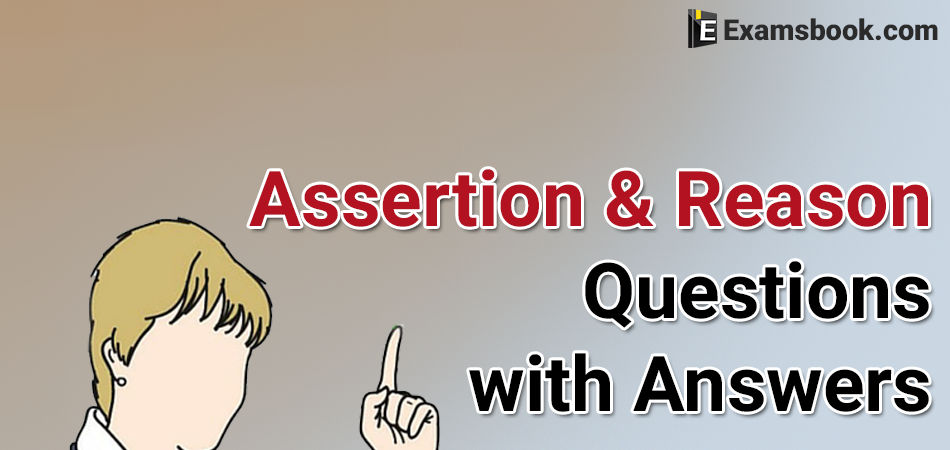• Save

# Assertion and Reason Questions with Answers for Competitive Exams

Vikram SinghLast year 23.4K ViewsHere I am sharing you assertion and reason questions with answers which is an important topic in the competitive exams. Assertion means a statement and reason means an explanation about that particular statement.

Important Questions of Assertion and Reason

Direction (1 to 5): In each of the questions given below, there are two statements marked as Assertion (A) and Reason (R). Mark your answer as per the codes provided below:

Q.1. Assertion (A):

It's colder on mountains than it is on plains.

Reason (R):

When altitude decreases, so does the temperature.

(A) A is true but R is false.

(B) Both A and R are true and R is the correct explanation of A.

(C) A is false but R is true.

(D) Both A and R are true but R is not the correct explanation of A.

(E) Both A and R are false.

Ans .   B

Q.2. Assertion (A):

When lightning strikes, the sound is heard a little after the flash is seen.

Reason (R):

The velocity of light is greater than that of the sound.

(A) A is true but R is false.

(B) Both A and R are true and R is the correct explanation of A.

(C) A is false but R is true.

(D) Both A and R are true but R is not the correct explanation of A.

(E) Both A and R are false.

Ans .   B

Q.3. Assertion (A):

Inside the earth metals are present in molten state.

Reason (R):

Earth absorbs the sun's rays.

(A) A is true but R is false.

(B) Both A and R are true and R is the correct explanation of A.

(C) A is false but R is true.

(D) Both A and R are true but R is not the correct explanation of A.

(E) Both A and R are false.

Ans .   A

Q.4. Assertion (A):

A ship rises as it enters the sea from a river.

Reason (R):

The density of sea water is higher as compared to river water.

(A) A is true but R is false.

(B) Both A and R are true and R is the correct explanation of A.

(C) A is false but R is true.

(D) Both A and R are true but R is not the correct explanation of A.

(E) Both A and R are false.

Ans .   B

Q.5. Assertion (A):

AIDS does not have a vaccine.

Reason (R):

The AIDS virus alters its genetic code.

(A) A is true but R is false.

(B) Both A and R are true and R is the correct explanation of A.

(C) A is false but R is true.

(D) Both A and R are true but R is not the correct explanation of A.

(E) Both A and R are false.

Ans .   B

Direction (6 to 10): In each of the questions given below, there are two statements marked as Assertion (A) and Reason (R). Mark your answer as per the codes provided below:

Q.6. Assertion (A):

Indian President is the head of the State.

Reason (R):

Indian Parliament consists of the President, Lok Sabha and Rajya Sabha.

(A) A is true but R is false.

(B) Both A and R are true and R is the correct explanation of A.

(C) A is false but R is true.

(D) Both A and R are true but R is not the correct explanation of A.

(E) Both A and R are false.

Ans .   D

Q.7. Assertion (A):

Clothes are not washed properly in hot water.

Reason (R):

Hard water contains many minerals.

(A) A is true but R is false.

(B) Both A and R are true and R is the correct explanation of A.

(C) A is false but R is true.

(D) Both A and R are true but R is not the correct explanation of A.

(E) Both A and R are false.

Ans .   D

Q.8. Assertion (A):

The British sovereignty continued to exist in f India.

Reason (R):

The British sovereign appointed the last Governor-General of free India

(A) A is true but R is false.

(B) Both A and R are true and R is the correct explanation of A.

(C) A is false but R is true.

(D) Both A and R are true but R is not the correct explanation of A.

(E) Both A and R are false.

Ans .   C

Q.9. Assertion (A):

In India, females have higher life expectancy than the males.

Reason (R):

(A) A is true but R is false.

(B) Both A and R are true and R is the correct explanation of A.

(C) A is false but R is true.

(D) Both A and R are true but R is not the correct explanation of A.

(E) Both A and R are false.

Ans .   E

Q.10. Assertion (A):

Sprouting should not be done before consuming the grains.

Reason (R):

Sprouting kills many vital vitamins.

(A) A is true but R is false.

(B) Both A and R are true and R is the correct explanation of A.

(C) A is false but R is true.

(D) Both A and R are true but R is not the correct explanation of A.

(E) Both A and R are false.

Ans .   D

If you have any doubt or want to ask anything related assertion and reason questions, you can ask me in the comment section. Go to the next page for more practice.

### Vikram Singh

Providing knowledgable questions of Reasoning and Aptitude for the competitive exams.